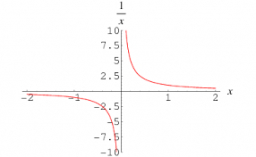# The product 5

The product of two numbers is 250. One factor is 1/10 of 250.
What is the second factor?
What is the reciprocal of 1/10 of 250?

b =  10
x =  0.04

### Step-by-step explanation:Did you find an error or inaccuracy? Feel free to write us. Thank you!

Tips to related online calculators
Need help to calculate sum, simplify or multiply fractions? Try our fraction calculator.